Next: Spurious switching activity (SSA) Up: Observations Previous: Observations

### Interconnect power consumption

We evaluated eight high-level synthesis benchmarks implemented in 0.18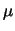m technology. Chemical and IIR77 are IIR filters used in the industry. DCT_IJPEG is the Independent JPEG Group's implementation of digital cosine transform (DCT) . DCT_Wang is the DCT algorithm named after the inventor . Both DCT algorithms work on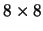pixels. Elliptic, an elliptic wave filter, and Diffeq, a differential equation solver, are from the NCSU CBL high-level synthesis benchmark suite . Jacobi is the Jacobi iterative algorithm for solving a fourth order linear system . WDF is an FIR wave digital filter. Table I gives the number of basic operations in the benchmark behaviors. It also indicates whether there are loops inside the behavior. The smallest benchmark is Diffeq with six multiplications, two additions and two subtractions. The largest is Jacobi with 24 multiplications, eight divisions, eight additions and 16 subtractions.
 Chemical DCT_IJPEG DCT_Wang Diffeq Elliptic IIR77 Jacobi WDF No. of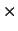17 12 21 6 0 22 24 8 No. of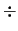0 0 0 0 0 0 8 0 No. of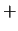9 24 14 2 26 14 8 26 No. of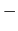6 12 12 2 0 0 16 0 Loop(Y/N) Y N N Y Y Y N Y

Using wire and gate capacitances data from , Fig. 1 shows the input capacitance of a minimum-width gate along with the capacitance (as the sum of area, fringing and coupling capacitance) of a minimum-width metal-1 wire of length of 20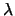(whereis the minimum feature size) for different DSM technologies. The figure shows that the two are comparable for current and future technologies. In a circuit, a data transfer wire between datapath units can be hundreds oflong, which is equivalent to tens of gates in terms of input capacitance. Its power consumption is thus non-negligible.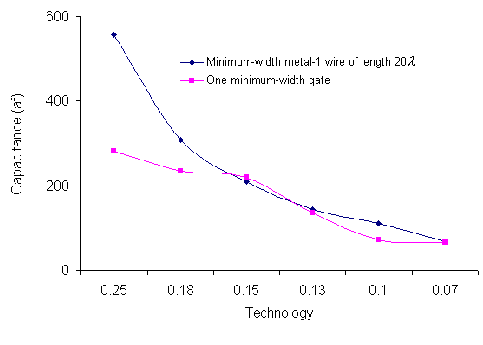We used the wire capacitances from the same source along with an RTL design library from NEC [44,45] to estimate power consumption for the benchmarks. The NEC RTL library was characterized for power and area through logic-level power and area estimation, which was verified to be within 10% of SPICE simulation. Fig. 2 shows the percentage of total power consumed by RTL interconnects for area-optimized (AO) and interconnect-unaware power-optimized (IUPO) benchmarks, respectively.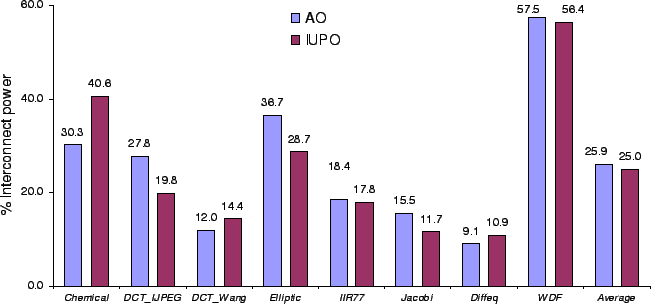In both AO and IUPO circuits, interconnects consume a significant percentage of total power, with the average percentage being 25.9% and 25.0%, respectively. It is worth noting that if the coupling effect is not considered, the interconnect power percentage will be 16.2% and 14.3%, respectively.Next: Spurious switching activity (SSA) Up: Observations Previous: Observations
Lin Zhong 2003-10-11